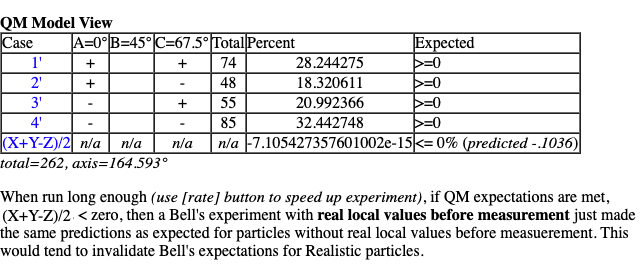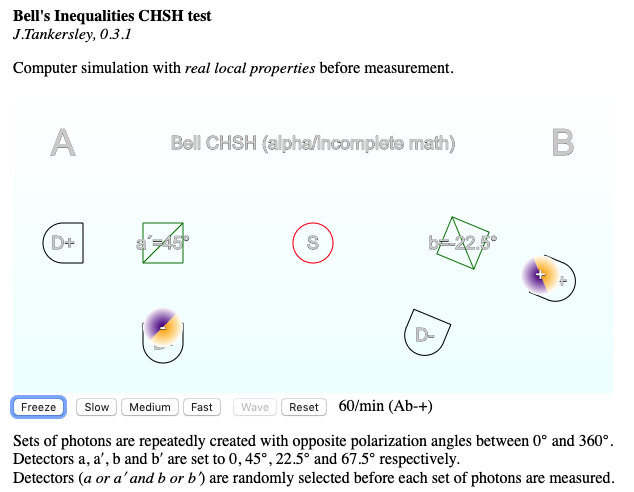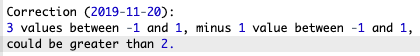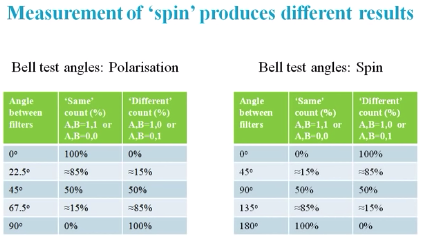## Does Bells Inequalities with Easy-Math expose a flaw?

Foundations of physics and/or philosophy of physics, and in particular, posts on unresolved or controversial issues

### Does Bells Inequalities with Easy-Math expose a flaw?

If the paper "Bell's Theorem with Easy Math, David R. Schneider" (https://drchinese.com/David/Bell_Theorem_Easy_Math.htm) is correct, then does it expose a flaw with Bell's Inequalities test, only allowing 1 possible result, regardless of any assumptions about particle properties?

Argument demonstrated with a simple simulation at https://codeserver.net/nb/
jtankersley

Posts: 18
Joined: Sun Nov 10, 2019 4:47 pm
Location: Middleton, WI USA

### Re: Does Bells Inequalities with Easy-Math expose a flaw?

The basic argument from https://codeserver.net/nb/ for review/criticism...

The simulation is for graphic demonstration, but the math argument (correct or not, based on my understanding from Schneider's Easy-Math work) appears to be rather basic.

This simple-math simulation demonstrates that results of a simplified Bell's Inequality experiment must be the same, regardless if particles have 'real local properties' before measurement or not. If this simulation is an accurate representation of the fundamental math behind Bell's Inequalities, then related experiments must be non-conclusive (neither proving nor dis-proving the Einstein-Podolsky-Rosen argument it was designed to test)?

This computer simulation models a Bell's Inequality experiment based on a simplified version of Schneider's Bell's Theorem and Negative Probabilities [v]. We model repeated creation of 3 correlated (entangled) photons (a, b and c). Each set of photons is created with the same polarization angle (between 0 and 360 degrees), randomly set when the photon's are created (Einstein's deterministic model). We then measure the photons polarity as being within 180 degrees (or not) of each of 3 detectors which are set to 0, 45 and 67.5 degrees. We show that if the photons are within 180 degrees of detectors at 0 and 67.5 degrees, then they must also be within 180 degrees of the detector set at 45 degrees. Simple math tells us this must be true (if x is between 0-180 and 0+180, and x is also between 67.5-180 and 67.5+180, then x must also be between 45-180 and 45+180). However Bell's theorem (according to Schneider [iv][v]) apparently requires us to violate that simple math in order for Einstein's deterministic (or hidden variables) model to win.

[iv] Schneider https://drchinese.com/David/Bell_Theorem_Easy_Math.htm
[v] Schneider http://drchinese.com/David/Bell_Theorem ... lities.htm
jtankersley

Posts: 18
Joined: Sun Nov 10, 2019 4:47 pm
Location: Middleton, WI USA

### Re: Does Bells Inequalities with Easy-Math expose a flaw?

jtankersley wrote:If the paper "Bell's Theorem with Easy Math, David R. Schneider" (https://drchinese.com/David/Bell_Theorem_Easy_Math.htm) is correct, then does it expose a flaw with Bell's Inequalities test, only allowing 1 possible result, regardless of any assumptions about particle properties?

Argument demonstrated with a simple simulation at https://codeserver.net/nb/

The simulation at https://codeserver.net/nb/ does not simulate the experiment described at https://journals.aps.org/ppf/pdf/10.110 ... zika.1.195 . Bell's inequality is about the latter experiment. The essential difference is that Bell's inequality refers to an experiment where two particles are prepared and then observed.
Mikko

Posts: 155
Joined: Mon Feb 17, 2014 2:53 am

### Re: Does Bells Inequalities with Easy-Math expose a flaw?

Agreed that the simulation does not represent any specific Bell experiment.
It is a simplification, reducing to the simplest possible observable experiment, and simplest possible math.

This appears to be what Bell is actually testing for, according to Schneider.

Bell's Inequality test was supposed to prove or disprove the EPR (Einstein–Podolsky–Rosen) paper, but the requirements for EPR to win are not mathematically possible, based on Schneider "Bell Theorem Negative Probabilities" paper (i), (test cases 2 and 7).

So either I am mis-understanding Schneider, or Schneider is mis-understanding Bell, or Bell is wrong.

(i) Schneider, Bell Theorem Negative Probabilities http://drchinese.com/David/Bell_Theorem ... lities.htm
jtankersley

Posts: 18
Joined: Sun Nov 10, 2019 4:47 pm
Location: Middleton, WI USA

### Re: Does Bells Inequalities with Easy-Math expose a flaw?

Correction above: "if x is between 0-90 and 0+90, and x is also between 67.5-90 and 67.5+90, then x must also be between 45-90 and 45+90"
jtankersley

Posts: 18
Joined: Sun Nov 10, 2019 4:47 pm
Location: Middleton, WI USA

### Re: Does Bells Inequalities with Easy-Math expose a flaw?

jtankersley wrote:Agreed that the simulation does not represent any specific Bell experiment.
It is a simplification, reducing to the simplest possible observable experiment, and simplest possible math.

This appears to be what Bell is actually testing for, according to Schneider.

Bell's Inequality test was supposed to prove or disprove the EPR (Einstein–Podolsky–Rosen) paper, but the requirements for EPR to win are not mathematically possible, based on Schneider "Bell Theorem Negative Probabilities" paper (i), (test cases 2 and 7).

So either I am mis-understanding Schneider, or Schneider is mis-understanding Bell, or Bell is wrong.

(i) Schneider, Bell Theorem Negative Probabilities http://drchinese.com/David/Bell_Theorem ... lities.htm

If you look through some of the other threads here, you will find that Bell was definitely wrong. It is pretty easy. NOTHING can "violate" the Bell inequalities. It is both mathematically and physically impossible.
.
FrediFizzx
Independent Physics Researcher

Posts: 1743
Joined: Tue Mar 19, 2013 7:12 pm
Location: N. California, USA

### Re: Does Bells Inequalities with Easy-Math expose a flaw?

***
Bell's theorem is a fraud. Some of the followers of Bell are intelligent enough to recognize that it is a fraud but it is too late for them to admit that fact.For technical argument please see https://arxiv.org/abs/1704.02876.

***
Joy Christian
Research Physicist

Posts: 2201
Joined: Wed Feb 05, 2014 4:49 am
Location: Oxford, United Kingdom

### Re: Does Bells Inequalities with Easy-Math expose a flaw?

jtankersley wrote:Agreed that the simulation does not represent any specific Bell experiment. It is a simplification, reducing to the simplest possible observable experiment, and simplest possible math.

The "simulation" does not simulate any experiment. In particular, there is no source that could produced three photons as in the "simulation".
jtankersley wrote:This appears to be what Bell is actually testing for, according to Schneider.

Even if the experiment were possible, Schneider's page is about a different experiment, so this "simulation" is not relevant.
jtankersley wrote:Bell's Inequality test was supposed to prove or disprove the EPR (Einstein–Podolsky–Rosen) paper, but the requirements for EPR to win are not mathematically possible, based on Schneider "Bell Theorem Negative Probabilities" paper (i), (test cases 2 and 7).

Bell's article proved that Einstein & Podolsky & Rosen had failed to consider an important point.
jtankersley wrote: So either I am mis-understanding Schneider, or Schneider is mis-understanding Bell, or Bell is wrong.

That you are mis-understanding Schneider is obvious.
There is no reason to think that Schneider is mis-understanding Bell. In particular, the bold line in the introduction is correct. The rest does not depend on correct understanding of Bell. The math is different anyway, as indicated by the title, and can be checked independently of anything Bell has said.
Whether Bell is wrong is already answered (correctly or otherwise) by Joy Christian, who has worked on this point much more than I have.
Mikko

Posts: 155
Joined: Mon Feb 17, 2014 2:53 am

### Re: Does Bells Inequalities with Easy-Math expose a flaw?

Hello Mikko,

I am a fan of Joy Christian's work using advanced math to challenge Bell, but unfortunately I was unable to follow the math in the papers of his that I tried to read. Any recommended reading for understanding his work that does not require post-doctorate level math skills, perhaps good threads on this site would be appreciated.

What I can do though is write computer software, version 0.1 of the app was an incomplete draft, version 0.2 is more complete (but still beta) at http://codeserver.net/nb/.

This version now also calculates QM Expectations, simplified from Scheider, but I suspect possibly equivalent enough to be relevant.

What appeared somewhat interesting is that the results appear to be producing some (very small) negative values (and positive) for (X+Y-Z)/2 (for explanation see reference [I] on the app). But they appear to just be rounding errors (as I don't see much opportunity for counting or timing errors in the app which appear to be the only path I see for non-zero results).

Negative values for (X+Y-Z)/2 are the results expected for "QM Model View", but not if particles have "real local properties" before measurement, as they do in the app experiment. Or the negative result could indicate proof of a "pilot wave" in nature, as I am told Bell himself suspected I believe I read (but so far the math does not make sense to me as explained by Schneider. The (X+Y-Z)/2 calculation can be reduced to cases 1+4+5+8 - cases (1+4+5+8) once you remove the impossible cases 2 and 7, so how can this be anything but zero, except for rounding, counting or timing errors? I don't get it.)

In any event, it is relatively simple (for a computer programmer) to replicate the math in this experiment with "real local properties" using a computer program. Seemed like a good way to validate or invalidate Bell's expectations using "simple math" and "realistic models" that the average scientific person can easily understand, problem is, the math still does not appear to add up (what am I missing!).

Review, help and/or criticism welcome (politely please).^^^ appears to be rounding errors, briefly seemed interesting, now Bell's math leaves me confused as to how any experiment achieved negative values for the (X+Y-Z)/2 calculation, something must be missing...
jtankersley

Posts: 18
Joined: Sun Nov 10, 2019 4:47 pm
Location: Middleton, WI USA

### Re: Does Bells Inequalities with Easy-Math expose a flaw?

jtankersley wrote:What I can do though is write computer software, version 0.1 of the app was an incomplete draft, version 0.2 is more complete (but still beta) at http://codeserver.net/nb/.

This version now also calculates QM Expectations, simplified from Scheider, but I suspect possibly equivalent enough to be relevant.

The version 0.2 still makes no attempt to relate the "simulation" to the experiment discussed by Schneider. In particular, the correspondence to the figure 2 on the page http://www.drchinese.com/David/Bell_The ... lities.htm should be presented.

You should present the computed numbers as rounded to a reasonable number of decimals. E.g., toFixed(2) would be more that enough.

For a small number of cases the results cannot be very accurate. Therefore the angles should be 120° apart as on the page https://drchinese.com/David/Bell_Theorem_Easy_Math.htm for maximal effect.

But as long as your "simulation" has no connection to Bell's theorem and related experiments these details are rather unimportant.

The answer to the title question is that so far you have not found any flaw anywhere.
Mikko

Posts: 155
Joined: Mon Feb 17, 2014 2:53 am

### Re: Does Bells Inequalities with Easy-Math expose a flaw?

Hi Mikko,

Agreed that the simulation with Easy-Math does not appear to "expose a flaw". A more useful simulation would need to replicate actual experiments (pairs of particles) and use the same math (that apparently calculates "negative values" for cases 2 and 7 from actual experimental results).

That 'realistic model' simulation would likely produce nothing too interesting, just the same results Bell expects for a realistic model (no violation). But it may be more interesting if adding a simulated pilot wave could violate Bell's inequalities (apparently suggested in reference  below).
Working on learning how to do that now.

Questions:
What math is being used to calculate negative values for cases  and  and from what experimental results?
I would expect that a realistic model simulation (using non-simplified setup and non-simplified math) would not produce experimental results that calculate negative values for cases  and , but could a simulated 'pilot wave' of some sort cause the simulation to violate Bell's Inequalities (see reference  that appears to discuss/propose similar, based on fluid-mechanical wave experiments by John Bush of MIT)?
To be continued with a 'next version' of this experiment after further study/research and time permitting...Louis Vervoort, 05.04.2018, (University of Tyumen, Russia) Are Hidden-Variable Theories for Pilot-Wave Systems Possible ? https://arxiv.org/pdf/1701.08194.pdf
jtankersley

Posts: 18
Joined: Sun Nov 10, 2019 4:47 pm
Location: Middleton, WI USA

### Re: Does Bells Inequalities with Easy-Math expose a flaw?

Mikko wrote:
Bell's article proved that Einstein & Podolsky & Rosen had failed to consider an important point.

I disagree with this folklore. Einstein, Podolsky, and Rosen (EPR) did not fail in any sense. In fact, the EPR argument is a very powerful and logically impeccable argument.

Bell, on the contrary, made several subtle mistakes in his so-called "theorem." One of these mistakes by Bell and his followers is to over-apply the EPR criterion of reality.

In footnote 3, pager 2, of this paper I have explained, using a homely example, what went wrong in Bell's implementation of the EPR criterion of reality in his "theorem."

***
Joy Christian
Research Physicist

Posts: 2201
Joined: Wed Feb 05, 2014 4:49 am
Location: Oxford, United Kingdom

### Re: Does Bells Inequalities with Easy-Math expose a flaw?

jtankersley wrote:But it may be more interesting if adding a simulated pilot wave could violate Bell's inequalities

Even "pilot waves" cannot surpass the speed of light and thereby violate Lorentz invariance. So the idea is useless for arbitrary separation of the stations. If you want to talk instead about the "quantum potential" that is already nonlocal by definition.
local

Posts: 86
Joined: Mon Aug 05, 2019 1:19 pm

### Re: Does Bells Inequalities with Easy-Math expose a flaw?

I upgraded the (animated) simulation https://codeserver.net/bell/2/ to match a basic CHSH setup.

But I must be calculating this incorrectly, because the way I am doing it, it would be impossible to violate Bell's Inequality (would need negative values for cases  and , which I am generating as simple percents, experimental counts, no way to get "negative counts" the way I am doing it).

Example: How can (a-b-c+d)/(a+b+c+d) be >2 unless either b and/or c are negative? So must be a calculation I am missing.

I thought the next effort would be to see how adding simulated "pilot waves" could violate the in-equality, but first I'll have to learn what actual math is being used.

So the next effort may be to read Joy's paper https://arxiv.org/pdf/1704.02876.pdf, Thank you

https://codeserver.net/bell/2/jtankersley

Posts: 18
Joined: Sun Nov 10, 2019 4:47 pm
Location: Middleton, WI USA

### Re: Does Bells Inequalities with Easy-Math expose a flaw?

Correction, maths needed another level of calculation.
Not sure how close to correct it is, but it no longer appears to be impossible math (3 values between 0 and 1, minus 1 value between 0 and 1, could be greater than 2).Do we know if anyone/anywhere has tried a real-world Bell experiment where the photons are pre-measured before being sent to the polarizers and detectors, to prove the test would then fail Bell's inequalities?
(I know measuring single photons going through double slit experiments interferes, would be interesting to see if pre-measuring photon polarization would similarly interfere or not...)
jtankersley

Posts: 18
Joined: Sun Nov 10, 2019 4:47 pm
Location: Middleton, WI USA

### Re: Does Bells Inequalities with Easy-Math expose a flaw?

Has anyone seen this video https://www.youtube.com/watch?v=yOtsEgbg1-s by Karma Peny?jtankersley

Posts: 18
Joined: Sun Nov 10, 2019 4:47 pm
Location: Middleton, WI USA

### Re: Does Bells Inequalities with Easy-Math expose a flaw?

Another random stupid youtube video. It's just the old detection loophole. Single detectors at a side (rather than 2) together with the CH inequality and a detection efficiency above a threshold allow to avoid the loophole.
local

Posts: 86
Joined: Mon Aug 05, 2019 1:19 pm